Next: Definition: Up: PageRank Previous: PageRank   Contents   Index

## Markov chains

A Markov chain is a discrete-time stochastic process: a process that occurs in a series of time-steps in each of which a random choice is made. A Markov chain consists of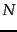states. Each web page will correspond to a state in the Markov chain we will formulate.

A Markov chain is characterized by an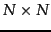transition probability matrix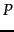each of whose entries is in the interval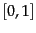; the entries in each row ofadd up to 1. The Markov chain can be in one of thestates at any given time-step; then, the entry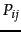tells us the probability that the state at the next time-step is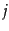, conditioned on the current state being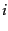. Each entryis known as a transition probability and depends only on the current state; this is known as the Markov property. Thus, by the Markov property,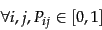(251)

and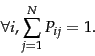(252)

A matrix with non-negative entries that satisfies Equation 252 is known as a stochastic matrix . A key property of a stochastic matrix is that it has a principal left eigenvector corresponding to its largest eigenvalue, which is 1.

In a Markov chain, the probability distribution of next states for a Markov chain depends only on the current state, and not on how the Markov chain arrived at the current state. Figure 21.2 shows a simple Markov chain with three states. From the middle state A, we proceed with (equal) probabilities of 0.5 to either B or C. From either B or C, we proceed with probability 1 to A. The transition probability matrix of this Markov chain is then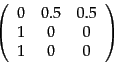(253)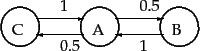A Markov chain's probability distribution over its states may be viewed as a probability vector : a vector all of whose entries are in the interval, and the entries add up to 1. An-dimensional probability vector each of whose components corresponds to one of thestates of a Markov chain can be viewed as a probability distribution over its states. For our simple Markov chain of Figure 21.2 , the probability vector would have 3 components that sum to 1.

We can view a random surfer on the web graph as a Markov chain, with one state for each web page, and each transition probability representing the probability of moving from one web page to another. The teleport operation contributes to these transition probabilities. The adjacency matrix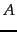of the web graph is defined as follows: if there is a hyperlink from pageto page, then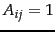, otherwise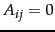. We can readily derive the transition probability matrixfor our Markov chain from thematrix:

1. If a row ofhas no 1's, then replace each element by 1/N. For all other rows proceed as follows.
2. Divide each 1 inby the number of 1's in its row. Thus, if there is a row with three 1's, then each of them is replaced by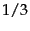.
3. Multiply the resulting matrix by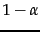.
4. Add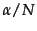to every entry of the resulting matrix, to obtain.

We can depict the probability distribution of the surfer's position at any time by a probability vector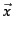. At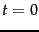the surfer may begin at a state whose corresponding entry inis 1 while all others are zero. By definition, the surfer's distribution at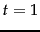is given by the probability vector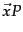; at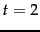by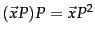, and so on. We will detail this process in Section 21.2.2 . We can thus compute the surfer's distribution over the states at any time, given only the initial distribution and the transition probability matrix.

If a Markov chain is allowed to run for many time steps, each state is visited at a (different) frequency that depends on the structure of the Markov chain. In our running analogy, the surfer visits certain web pages (say, popular news home pages) more often than other pages. We now make this intuition precise, establishing conditions under which such the visit frequency converges to fixed, steady-state quantity. Following this, we set the PageRank of each node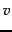to this steady-state visit frequency and show how it can be computed.

SubsectionsNext: Definition: Up: PageRank Previous: PageRank   Contents   Index